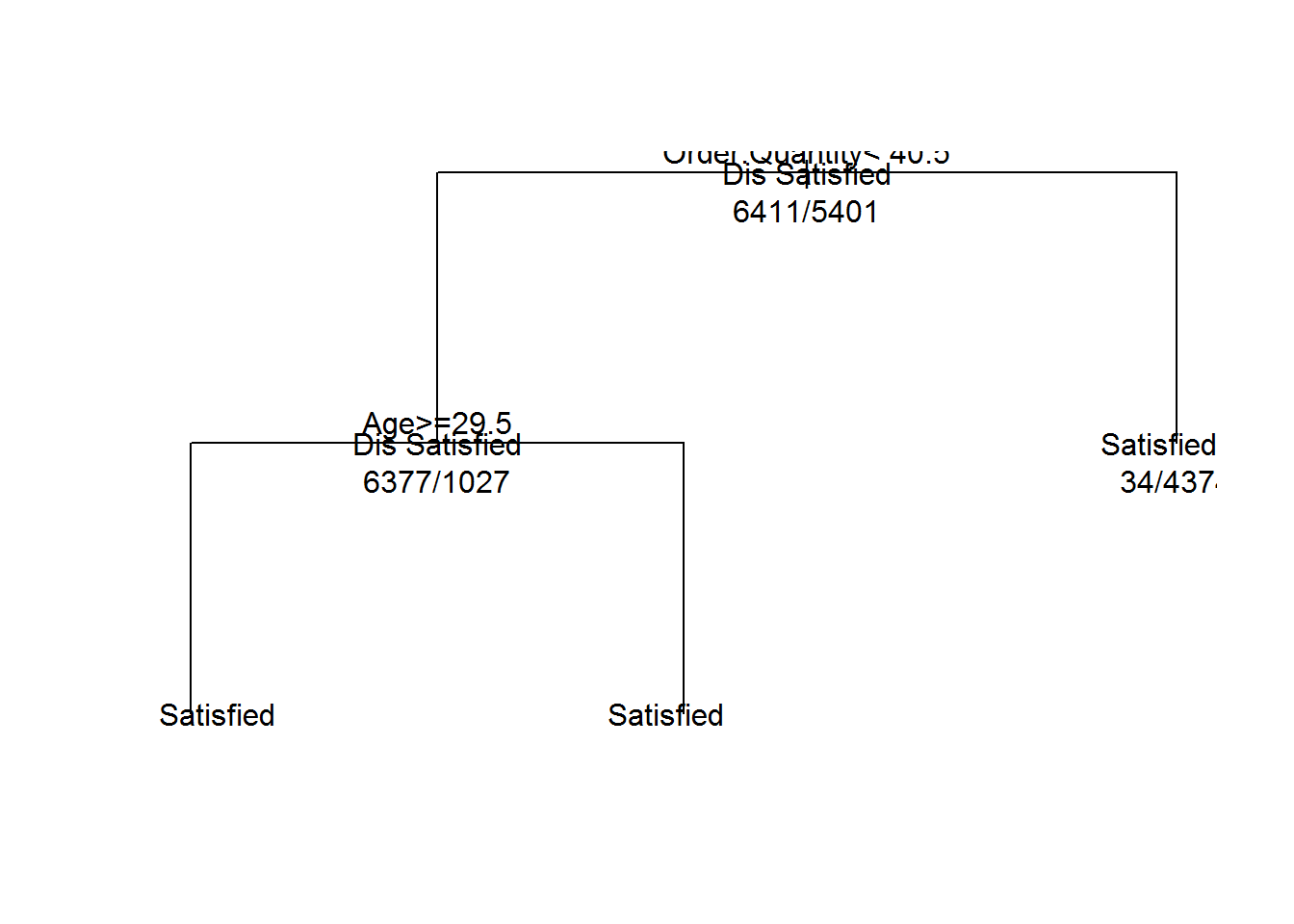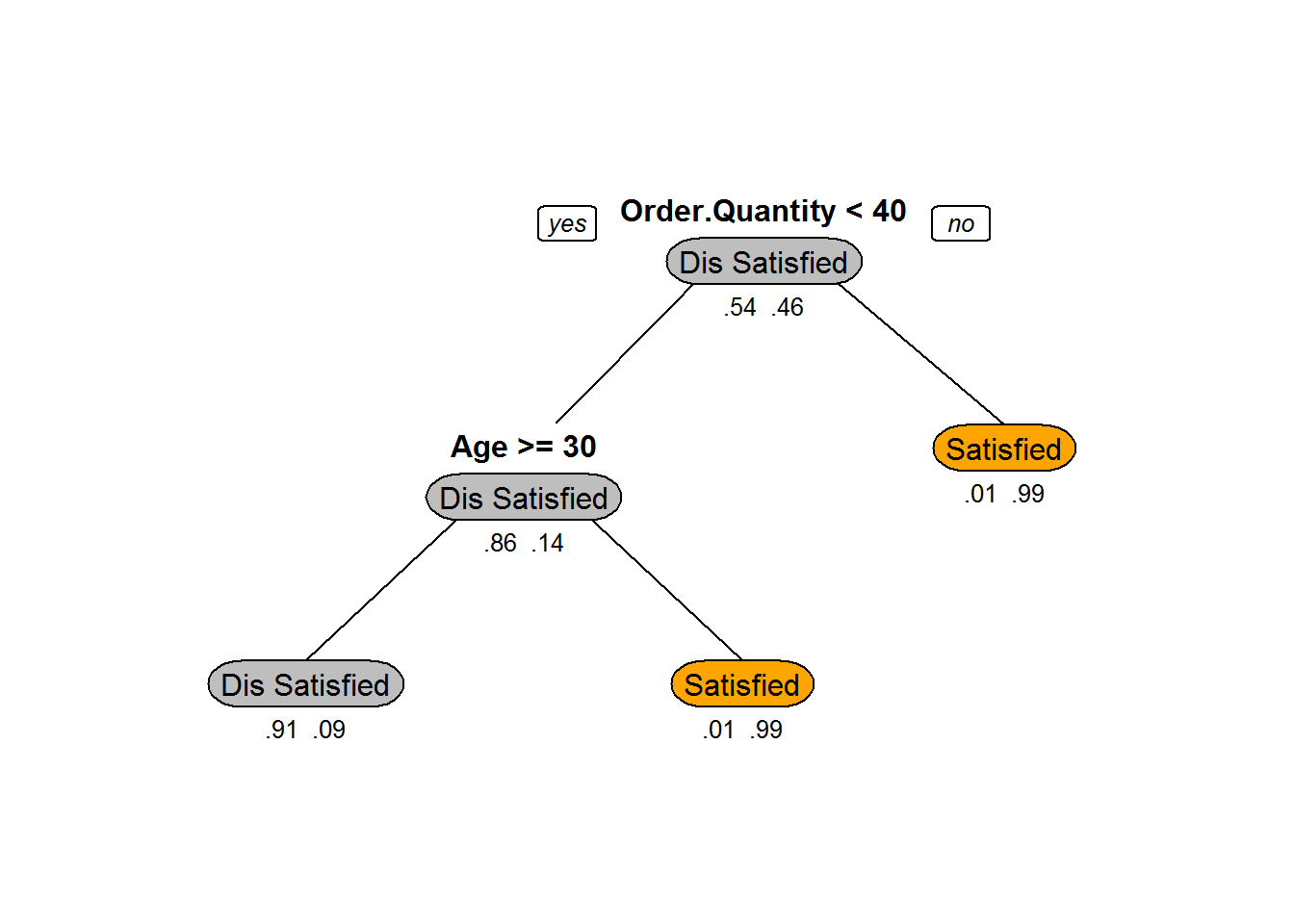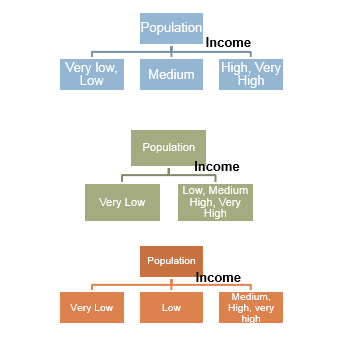• No products in the cart.

# 203.3.7 Building a Decision Tree in R

### LAB: Decision Tree Building

In previous section, we studied about Information Gain in Decision Tree Split

• Import Data:Ecom_Cust_Relationship_Management/Ecom_Cust_Survey.csv
• How many customers have participated in the survey?
• Overall most of the customers are satisfied or dis-satisfied?
• Can you segment the data and find the concentrated satisfied and dis-satisfied customer segments ?
• What are the major characteristics of satisfied customers?
• What are the major characteristics of dis-satisfied customers?

### Solution

Ecom_Cust_Survey <- read.csv("C:\\Amrita\\Datavedi\\Ecom_Cust_Relationship_Management\\Ecom_Cust_Survey.csv")
• How many customers have participated in the survey?
nrow(Ecom_Cust_Survey)
##  11812
• Overall most of the customers are satisfied or dis-satisfied?
table(Ecom_Cust_Survey$Overall_Satisfaction) ## ## Dis Satisfied Satisfied ## 6411 5401 ### Code-Decision Tree Building rpart(formula, method, data, control) • Formula : y~x1+x2+x3 • method: “Class” for classification trees , “anova” for regression trees with continuous output • For controlling tree growth. For example, control=rpart.control(minsplit=30, cp=0.001) • Minsplit : Minimum number of observations in a node be 30 before attempting a split • A split must decrease the overall lack of fit by a factor of 0.001 (cost complexity factor) before being attempted.(details later) • Need the library rpart library(rpart) • Building Tree Model Ecom_Tree<-rpart(Overall_Satisfaction~Region+ Age+ Order.Quantity+Customer_Type+Improvement.Area, method="class", data=Ecom_Cust_Survey) Ecom_Tree ## n= 11812 ## ## node), split, n, loss, yval, (yprob) ## * denotes terminal node ## ## 1) root 11812 5401 Dis Satisfied (0.542753132 0.457246868) ## 2) Order.Quantity< 40.5 7404 1027 Dis Satisfied (0.861291194 0.138708806) ## 4) Age>=29.5 7025 652 Dis Satisfied (0.907188612 0.092811388) * ## 5) Age< 29.5 379 4 Satisfied (0.010554090 0.989445910) * ## 3) Order.Quantity>=40.5 4408 34 Satisfied (0.007713249 0.992286751) * ### Plotting the Trees plot(Ecom_Tree, uniform=TRUE) text(Ecom_Tree, use.n=TRUE, all=TRUE)### A better looking tree library(rpart.plot) ## Warning: package 'rpart.plot' was built under R version 3.1.3 prp(Ecom_Tree,box.col=c("Grey", "Orange")[Ecom_Tree$frame\$yval],varlen=0, type=1,extra=4,under=TRUE)### Tree Validation• Accuracy=(TP+TN)/(TP+FP+FN+TN)
• Misclassification Rate=(FP+FN)/(TP+FP+FN+TN)

The next post is about Validating a Tree.

20th June 2017

### 0 responses on "203.3.7 Building a Decision Tree in R"

Statinfer Software Solutions LLP

Software Technology Parks of India,
NH16, Krishna Nagar, Benz Circle,